# Point Min Max x coordinate

## Recommended PostsHow to get a point from the list with the largest or smallest x-coordinate.

Example list of points.

`'((1.0 0.0) (2.2 2.0) (-2.0 0.0) ...)`

##### Share on other sitesOne way :

```(apply 'max (mapcar 'car '((1.0 0.0) (2.2 2.0) (-2.0 0.0))))

(apply 'min (mapcar 'car '((1.0 0.0) (2.2 2.0) (-2.0 0.0))))
```

##### Share on other sitesAnother to sort the list from bigger to smaller for the max and visa versa .

Max eg.

```(car (vl-sort (mapcar 'car '((1.0 0.0) (2.2 2.0) (-2.0 0.0))) '>))
```

Min eg.

```(car (vl-sort (mapcar 'car '((1.0 0.0) (2.2 2.0) (-2.0 0.0))) '<))
```

##### Share on other sites##### Share on other sitesYou're welcome anytime.

##### Share on other sitesYour code is very good but I need to get the x and y coordinate

Returns' (xmin y).

##### Share on other sitesYour code is very good but I need to get the x and y coordinate

Just replace the function car with cadr in my first example to get y coordinates .

##### Share on other sitesShould the result be a member of the list?

I.e. For a list of points:

`((1 4 0) (3 2 0) (4 5 0))`

Should the function return (1 4 0) or (1 2 0)?

##### Share on other sitesok geniusses , now you're all awake , some time ago i wrote this to sort a selectionset by coordinates. But i stink it can shorter en more intelligent (but that's obvious in my case ;-)

```

;el = elist , xl = x , yl = y , ml = matrix , sl = sorted elist
(defun LG_SortSelectionSet ( ss / e el i xl yl ml sl)
(if (and ss (> (sslength ss) 1)(setq i 0))
(progn
;ss -> elist ( ((ip)e1) ((ip)e2) .. )
(while (setq e (ssname ss i))
(setq el (append el (list (list (getip e) e))) i (1+ i)))
(setq xl (vl-sort (rdup (mapcar 'caar el)) '<)
yl (vl-sort (rdup (mapcar 'cadar el)) '>))
(foreach y yl (foreach x xl (setq ml (append ml (list (list x y))))))
(setq sl (no-nil (mapcar '(lambda (x) (if (assoc x el)(cadr (assoc x el)))) ml))))))

(defun rdup ( i / o );remove duplicates
(vl-remove-if '(lambda (x) (cond ((vl-position x o) t) ((setq o (cons x o)) nil))) i))

(defun getip (e);get insertionpoint

(defun no-nil (lst) (apply 'append (subst nil (list nil) (mapcar 'list lst))))
```

in order to keep the first code more readable i created a couple of little subs like rdup etc (yes I know , vanilla)

What is does is first make a list of all x coordinates , same for y , then remove duplicates , sort the list and then see which combinations actually excist in selection set. It does work but i suspect there must be a more efficient way. (right now i am a little bit lazy by asking you...)

gr. Rlx

Edited by rlx

##### Share on other sitesList:

'((1 4 0) (3 2 0) (4 5 0))

Result min x coordinate:

'(1 4 0)

##### Share on other sites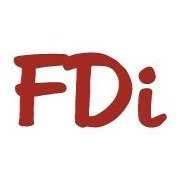I use something like this a lot for automated dimensions for line drawings. It maybe of some use :

```[b][color=BLACK]([/color][/b]defun c:linebs [b][color=FUCHSIA]([/color][/b]/ ss i en ed p1 p2 pl rl pt
minx miny maxx maxy
minxminy minxmaxy maxxminy maxxmaxy
minyminx minymaxx maxyminx maxymaxx[b][color=FUCHSIA])[/color][/b]

[b][color=FUCHSIA]([/color][/b]defun massoc [b][color=NAVY]([/color][/b]key alist / nlist[b][color=NAVY])[/color][/b]
[b][color=NAVY]([/color][/b]foreach x alist
[b][color=MAROON]([/color][/b]if [b][color=GREEN]([/color][/b]eq key [b][color=BLUE]([/color][/b]car x[b][color=BLUE])[/color][/b][b][color=GREEN])[/color][/b]
[b][color=GREEN]([/color][/b]setq nlist [b][color=BLUE]([/color][/b]cons [b][color=RED]([/color][/b]cdr x[b][color=RED])[/color][/b] nlist[b][color=BLUE])[/color][/b][b][color=GREEN])[/color][/b][b][color=MAROON])[/color][/b][b][color=NAVY])[/color][/b]
[b][color=NAVY]([/color][/b]reverse nlist[b][color=NAVY])[/color][/b][b][color=FUCHSIA])[/color][/b]

[b][color=FUCHSIA]([/color][/b]defun rnd_gvtol [b][color=NAVY]([/color][/b]value[b][color=NAVY])[/color][/b]
[b][color=NAVY]([/color][/b]* gv_tol [b][color=MAROON]([/color][/b]fix [b][color=GREEN]([/color][/b]/ [b][color=BLUE]([/color][/b][b][color=RED]([/color][/b]if [b][color=PURPLE]([/color][/b]minusp value[b][color=PURPLE])[/color][/b] - +[b][color=RED])[/color][/b] value [b][color=RED]([/color][/b]* gv_tol 0.5[b][color=RED])[/color][/b][b][color=BLUE])[/color][/b] gv_tol[b][color=GREEN])[/color][/b][b][color=MAROON])[/color][/b][b][color=NAVY])[/color][/b][b][color=FUCHSIA])[/color][/b]

[b][color=FUCHSIA]([/color][/b]setq gv_tol 0.001[b][color=FUCHSIA])[/color][/b][color=#8b4513];;GLOBAL TOLERANCE VALUE[/color]

[b][color=FUCHSIA]([/color][/b]and [b][color=NAVY]([/color][/b]setq ss [b][color=MAROON]([/color][/b]ssget [color=#2f4f4f]"X"[/color] [b][color=GREEN]([/color][/b]list [b][color=BLUE]([/color][/b]cons 0 [color=#2f4f4f]"LINE"[/color][b][color=BLUE])[/color][/b][b][color=BLUE]([/color][/b]cons 8 [b][color=RED]([/color][/b]getvar [color=#2f4f4f]"CLAYER"[/color][b][color=RED])[/color][/b][b][color=BLUE])[/color][/b][b][color=GREEN])[/color][/b][b][color=MAROON])[/color][/b][b][color=NAVY])[/color][/b]
[b][color=NAVY]([/color][/b]setq i 0[b][color=NAVY])[/color][/b]
[b][color=NAVY]([/color][/b]while [b][color=MAROON]([/color][/b]setq en [b][color=GREEN]([/color][/b]ssname ss i[b][color=GREEN])[/color][/b][b][color=MAROON])[/color][/b]
[b][color=MAROON]([/color][/b]setq ed [b][color=GREEN]([/color][/b]entget en[b][color=GREEN])[/color][/b]
p1 [b][color=GREEN]([/color][/b]list [b][color=BLUE]([/color][/b]rnd_gvtol [b][color=RED]([/color][/b]cadr [b][color=PURPLE]([/color][/b]assoc 10 ed[b][color=PURPLE])[/color][/b][b][color=RED])[/color][/b][b][color=BLUE])[/color][/b]
pl [b][color=GREEN]([/color][/b]cons p1 pl[b][color=GREEN])[/color][/b]
rl [b][color=GREEN]([/color][/b]cons [b][color=BLUE]([/color][/b]reverse p1[b][color=BLUE])[/color][/b] rl[b][color=GREEN])[/color][/b]
p2 [b][color=GREEN]([/color][/b]list [b][color=BLUE]([/color][/b]rnd_gvtol [b][color=RED]([/color][/b]cadr [b][color=PURPLE]([/color][/b]assoc 11 ed[b][color=PURPLE])[/color][/b][b][color=RED])[/color][/b][b][color=BLUE])[/color][/b]
pl [b][color=GREEN]([/color][/b]cons p2 pl[b][color=GREEN])[/color][/b]
rl [b][color=GREEN]([/color][/b]cons [b][color=BLUE]([/color][/b]reverse p2[b][color=BLUE])[/color][/b] rl[b][color=GREEN])[/color][/b][b][color=MAROON])[/color][/b]
[b][color=MAROON]([/color][/b]setq i [b][color=GREEN]([/color][/b]1+ i[b][color=GREEN])[/color][/b][b][color=MAROON])[/color][/b][b][color=NAVY])[/color][/b][b][color=FUCHSIA])[/color][/b]

[b][color=FUCHSIA]([/color][/b]setq minx [b][color=NAVY]([/color][/b]apply 'min [b][color=MAROON]([/color][/b]mapcar 'car pl[b][color=MAROON])[/color][/b][b][color=NAVY])[/color][/b]
maxx [b][color=NAVY]([/color][/b]apply 'max [b][color=MAROON]([/color][/b]mapcar 'car pl[b][color=MAROON])[/color][/b][b][color=NAVY])[/color][/b]
miny [b][color=NAVY]([/color][/b]apply 'min [b][color=MAROON]([/color][/b]mapcar 'car rl[b][color=MAROON])[/color][/b][b][color=NAVY])[/color][/b]
maxy [b][color=NAVY]([/color][/b]apply 'max [b][color=MAROON]([/color][/b]mapcar 'car rl[b][color=MAROON])[/color][/b][b][color=NAVY])[/color][/b]
minyminx [b][color=NAVY]([/color][/b]apply 'min [b][color=MAROON]([/color][/b]mapcar 'car [b][color=GREEN]([/color][/b]massoc minx pl[b][color=GREEN])[/color][/b][b][color=MAROON])[/color][/b][b][color=NAVY])[/color][/b]
maxyminx [b][color=NAVY]([/color][/b]apply 'max [b][color=MAROON]([/color][/b]mapcar 'car [b][color=GREEN]([/color][/b]massoc minx pl[b][color=GREEN])[/color][/b][b][color=MAROON])[/color][/b][b][color=NAVY])[/color][/b]
minymaxx [b][color=NAVY]([/color][/b]apply 'min [b][color=MAROON]([/color][/b]mapcar 'car [b][color=GREEN]([/color][/b]massoc maxx pl[b][color=GREEN])[/color][/b][b][color=MAROON])[/color][/b][b][color=NAVY])[/color][/b]
maxymaxx [b][color=NAVY]([/color][/b]apply 'max [b][color=MAROON]([/color][/b]mapcar 'car [b][color=GREEN]([/color][/b]massoc maxx pl[b][color=GREEN])[/color][/b][b][color=MAROON])[/color][/b][b][color=NAVY])[/color][/b]
minxminy [b][color=NAVY]([/color][/b]apply 'min [b][color=MAROON]([/color][/b]mapcar 'car [b][color=GREEN]([/color][/b]massoc miny rl[b][color=GREEN])[/color][/b][b][color=MAROON])[/color][/b][b][color=NAVY])[/color][/b]
maxxminy [b][color=NAVY]([/color][/b]apply 'max [b][color=MAROON]([/color][/b]mapcar 'car [b][color=GREEN]([/color][/b]massoc miny rl[b][color=GREEN])[/color][/b][b][color=MAROON])[/color][/b][b][color=NAVY])[/color][/b]
minxmaxy [b][color=NAVY]([/color][/b]apply 'min [b][color=MAROON]([/color][/b]mapcar 'car [b][color=GREEN]([/color][/b]massoc maxy rl[b][color=GREEN])[/color][/b][b][color=MAROON])[/color][/b][b][color=NAVY])[/color][/b]
maxxmaxy [b][color=NAVY]([/color][/b]apply 'max [b][color=MAROON]([/color][/b]mapcar 'car [b][color=GREEN]([/color][/b]massoc maxy rl[b][color=GREEN])[/color][/b][b][color=MAROON])[/color][/b][b][color=NAVY])[/color][/b][b][color=FUCHSIA])[/color][/b]

[b][color=FUCHSIA]([/color][/b]foreach v [b][color=NAVY]([/color][/b]list [b][color=MAROON]([/color][/b]list [color=#2f4f4f]"minx"[/color] [color=#2f4f4f]"miny"[/color][b][color=MAROON])[/color][/b][b][color=MAROON]([/color][/b]list [color=#2f4f4f]"minx"[/color] [color=#2f4f4f]"maxy"[/color][b][color=MAROON])[/color][/b]
[b][color=MAROON]([/color][/b]list [color=#2f4f4f]"maxx"[/color] [color=#2f4f4f]"miny"[/color][b][color=MAROON])[/color][/b][b][color=MAROON]([/color][/b]list [color=#2f4f4f]"maxx"[/color] [color=#2f4f4f]"maxy"[/color][b][color=MAROON])[/color][/b]
[b][color=MAROON]([/color][/b]list [color=#2f4f4f]"minx"[/color] [color=#2f4f4f]"minyminx"[/color][b][color=MAROON])[/color][/b][b][color=MAROON]([/color][/b]list [color=#2f4f4f]"minx"[/color] [color=#2f4f4f]"maxyminx"[/color][b][color=MAROON])[/color][/b]
[b][color=MAROON]([/color][/b]list [color=#2f4f4f]"maxx"[/color] [color=#2f4f4f]"minymaxx"[/color][b][color=MAROON])[/color][/b][b][color=MAROON]([/color][/b]list [color=#2f4f4f]"maxx"[/color] [color=#2f4f4f]"maxymaxx"[/color][b][color=MAROON])[/color][/b]
[b][color=MAROON]([/color][/b]list [color=#2f4f4f]"minxminy"[/color] [color=#2f4f4f]"miny"[/color][b][color=MAROON])[/color][/b][b][color=MAROON]([/color][/b]list [color=#2f4f4f]"maxxminy"[/color] [color=#2f4f4f]"miny"[/color][b][color=MAROON])[/color][/b]
[b][color=MAROON]([/color][/b]list [color=#2f4f4f]"minxmaxy"[/color] [color=#2f4f4f]"maxy"[/color][b][color=MAROON])[/color][/b][b][color=MAROON]([/color][/b]list [color=#2f4f4f]"maxxmaxy"[/color] [color=#2f4f4f]"maxy"[/color][b][color=MAROON])[/color][/b][b][color=NAVY])[/color][/b]

[b][color=NAVY]([/color][/b]entmake [b][color=MAROON]([/color][/b]list [b][color=GREEN]([/color][/b]cons 0 [color=#2f4f4f]"TEXT"[/color][b][color=GREEN])[/color][/b][b][color=GREEN]([/color][/b]cons 10 pt[b][color=GREEN])[/color][/b][b][color=GREEN]([/color][/b]cons 11 pt[b][color=GREEN])[/color][/b]
[b][color=GREEN]([/color][/b]cons 1 [b][color=BLUE]([/color][/b]strcat [b][color=RED]([/color][/b]car v[b][color=RED])[/color][/b] [color=#2f4f4f]","[/color] [b][color=RED]([/color][/b]cadr v[b][color=RED])[/color][/b][b][color=BLUE])[/color][/b][b][color=GREEN])[/color][/b]
[b][color=GREEN]([/color][/b]cons 7 [b][color=BLUE]([/color][/b]getvar [color=#2f4f4f]"TEXTSTYLE"[/color][b][color=BLUE])[/color][/b][b][color=GREEN])[/color][/b]
[b][color=GREEN]([/color][/b]cons 40 1.8[b][color=GREEN])[/color][/b][b][color=GREEN]([/color][/b]cons 41 0.9[b][color=GREEN])[/color][/b]
[b][color=GREEN]([/color][/b]cons 62 1[b][color=GREEN])[/color][/b][b][color=GREEN]([/color][/b]cons 72 4[b][color=GREEN])[/color][/b][b][color=MAROON])[/color][/b][b][color=NAVY])[/color][/b][b][color=FUCHSIA])[/color][/b]
[b][color=FUCHSIA]([/color][/b]prin1[b][color=FUCHSIA])[/color][/b][b][color=BLACK])[/color][/b]
```

-David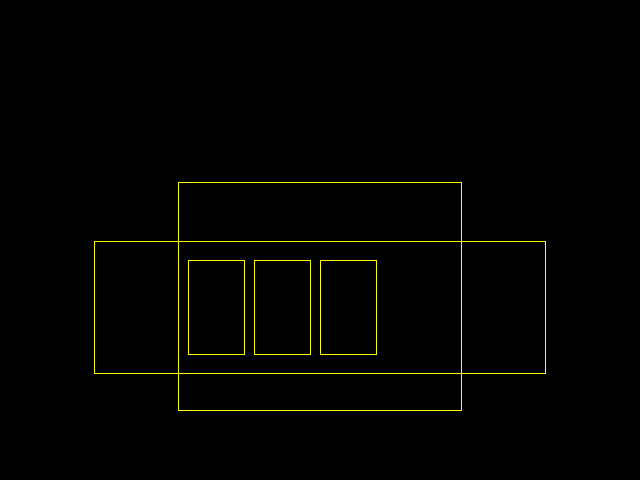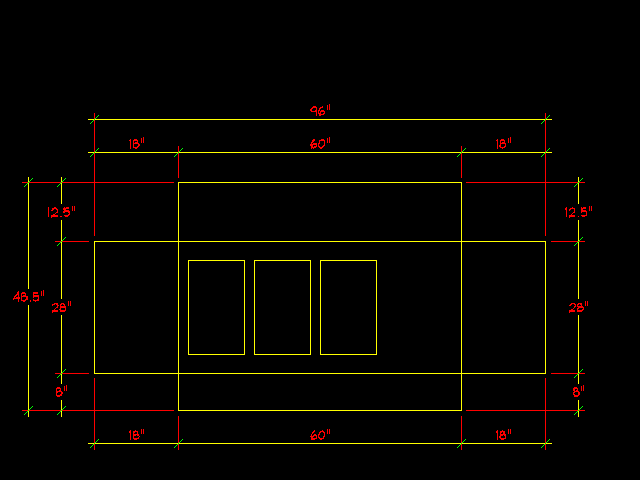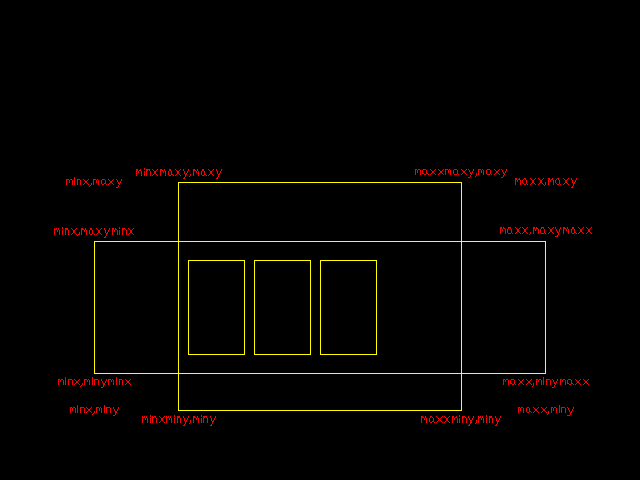TEST.DWG

##### Share on other sitesList:

'((1 4 0) (3 2 0) (4 5 0))

Result min x coordinate:

'(1 4 0)

A few ways to tackle this:

```(defun f1 ( l )
(car (vl-sort l '(lambda ( a b ) (< (car a) (car b)))))
)

_\$ (f1 '((1 4 0) (3 2 0) (4 5 0)))
(1 4 0)```

```(defun f2 ( l / r )
(setq r (car l))
(foreach x (cdr l) (if (< (car x) (car r)) (setq r x)))
r
)

_\$ (f2 '((1 4 0) (3 2 0) (4 5 0)))
(1 4 0)```

```(defun f3 ( l )
(if (cdr l)
(if (< (caar l) (caadr l))
(f3 (cons (car l) (cddr l)))
(f3 (cdr l))
)
(car l)
)
)

_\$ (f3 '((1 4 0) (3 2 0) (4 5 0)))
(1 4 0)```

##### Share on other sitesWhat is does is first make a list of all x coordinates , same for y , then remove duplicates , sort the list and then see which combinations actually excist in selection set.

The output would depend on whether you are looking to sort by x-coordinate and then by y-coordinate (or vice-versa), or whether you are looking to sort by distance from the origin.

For the latter, I would suggest something along the lines of:

```(defun sortselset ( s / e i l p )
(repeat (setq i (sslength s))
(setq e (ssname s (setq i (1- i)))
p (cons (cdr (assoc 10 (entget e))) p)
l (cons e l)
)
)
(mapcar
'(lambda ( n ) (nth n l))
(vl-sort-i p '(lambda ( a b ) (< (distance '(0 0) a) (distance '(0 0) b))))
)
)```

##### Share on other sitesA generic sorting function is likely more useful, e.g. sorting by x-coordinate:

```(defun sortselset ( s f / e i l p )
(repeat (setq i (sslength s))
(setq e (ssname s (setq i (1- i)))
p (cons (cdr (assoc 10 (entget e))) p)
l (cons e l)
)
)
(mapcar '(lambda ( n ) (nth n l)) (vl-sort-i p f))
)```

`(sortselset <selection-set> '(lambda ( a b ) (< (car a) (car b))))`

##### Share on other sitesI will have a look at your code Lee , but not now : weekend! :-)

the natural way is up-down , left-right so that is what I built in.

thanx

##### Share on other sitesI will have a look at your code Lee , but not now : weekend! :-)

You're welcome!the natural way is up-down , left-right so that is what I built in.

For up-down/left-right:

```(sortselset <selection-set>
'(lambda ( a b )
(< (car  a) (car  b))
)
)
)```

##### Share on other sitesYou're welcome!For up-down/left-right:

```(sortselset <selection-set>
'(lambda ( a b )
(< (car  a) (car  b))
)
)
)```

looks like exactly what the dragon , pardon , doctor ordered , thanx Lee , real smooth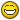##### Share on other siteshello David, you could upload the original routine linebs Thanks

##### Share on other siteshello David, you could upload the original routine linebs Thanks

This is a stand alone auto_dimension of a sort.

These ARE NOT associative DIMENSION entities.

There are several personal settings that can be manipulated to your tastes

```(defun c:minmax (/ ss i en ed p pl rl hf vf tz minx miny maxx maxy
minxminy minxmaxy maxxminy maxxmaxy
minyminx minymaxx maxyminx maxymaxx
ll lr ur ul llx lly lrx lry urx ury ulx uly)
;(trace dim_auto)

(defun massoc (key alist / nlist)
(foreach x alist
(if (eq key (car x))
(setq nlist (cons (cdr x) nlist))))
(reverse nlist))

(defun round (value to)
(setq to (abs to))
(* to (fix (/ ((if (minusp value) - +) value (* to 0.5)) to))))

(if (not (setq ss (ssget "X" (list (cons 0 "LINE")(cons 8 (getvar "CLAYER"))))))
(alert (strcat "No LINEs Found On Current LAyer " (getvar "CLAYER")))
(progn
(setq i 0)
(while (setq en (ssname ss i))
(setq ed (entget en))
(foreach g '(10 11)
(setq p (list (round (cadr (assoc g ed)) 0.1)
(round (caddr (assoc g ed)) 0.1))
pl (cons p pl)
rl (cons (reverse p) rl)))
(setq i (1+ i)))

(setq minx (apply 'min (mapcar 'car pl))
maxx (apply 'max (mapcar 'car pl))
miny (apply 'min (mapcar 'car rl))
maxy (apply 'max (mapcar 'car rl))
minyminx (apply 'min (mapcar 'car (massoc minx pl)))
maxyminx (apply 'max (mapcar 'car (massoc minx pl)))
minymaxx (apply 'min (mapcar 'car (massoc maxx pl)))
maxymaxx (apply 'max (mapcar 'car (massoc maxx pl)))
minxminy (apply 'min (mapcar 'car (massoc miny rl)))
maxxminy (apply 'max (mapcar 'car (massoc miny rl)))
minxmaxy (apply 'min (mapcar 'car (massoc maxy rl)))
maxxmaxy (apply 'max (mapcar 'car (massoc maxy rl)))
ll (list minx miny 0)       lr (list maxx miny 0)
ur (list maxx maxy 0)       ul (list minx maxy 0)
llx (list minxminy miny 0)  lly (list minx minyminx 0)
lrx (list maxxminy miny 0)  lry (list maxx minymaxx 0)
urx (list maxxmaxy maxy 0)  ury (list maxx maxymaxx 0)
ulx (list minxminy maxy 0)  uly (list minx maxyminx 0))

(setq tz (getvar "TEXTSIZE")
vf (fix (* tz 4))
hf (fix (* tz 3)))

;;;ARG -> dim_auto "H"/"V" point1 point2 text_vector_point text_size

;;;TOP
(dim_auto "H" uly ulx (list 0 (+ maxy hf)) tz)
(dim_auto "H" ulx urx (list 0 (+ maxy hf)) tz)
(dim_auto "H" urx ury (list 0 (+ maxy hf)) tz)

;;;BOTTOM
(dim_auto "H" lly llx (list 0 (- miny hf)) tz)
(dim_auto "H" llx lrx (list 0 (- miny hf)) tz)
(dim_auto "H" lrx lry (list 0 (- miny hf)) tz)

;;;LEFT
(dim_auto "V" llx lly (list (- minx vf) 0) tz)
(dim_auto "V" lly uly (list (- minx vf) 0) tz)
(dim_auto "V" uly ulx (list (- minx vf) 0) tz)

;;;RIGHT
(dim_auto "V" lrx lry (list (+ maxx vf) 0) tz)
(dim_auto "V" lry ury (list (+ maxx vf) 0) tz)
(dim_auto "V" ury urx (list (+ maxx vf) 0) tz)

;;;OVERALL
(dim_auto "H" uly ury (list 0 (+ maxy hf hf)) tz)
(dim_auto "H" lly lry (list 0 (- miny hf hf)) tz)
(dim_auto "V" llx ulx (list (- minx vf vf) 0) tz)
(dim_auto "V" lrx urx (list (+ maxx vf vf) 0) tz)))

(prin1))

;;;ENTMAKE A SIMPLE DIMENSION BLOCK WITH ATTRIBUTES
;;;ARG -> dim_dir "H"/"V" point1 point2 text_vector_point text_size
;;;RET -> ename
(defun dim_auto (dr pt1 pt2 tp ts /
sp1 sp2 te1 te2 ofa scp
txv tcp lcp ep1 ep2 bn sdef
ara txs ta flg dsuf hs)

;;;PERSONALIZE SETTINGS
(setvar "DIMZIN"
(setvar "UNITMODE" 1)
(setvar "ELEVATION" 0.0)
(setvar "THICKNESS" 0)
(setvar "LUNITS" 2)
(setvar "LUPREC" 2)
(setq dsuf "\"")   ;;;DIMENSION SUFFIX ( Inch Mark )

(setq tp (list (round (car tp) 1)

(cond ((= dr "H")
(T                        (setq ta  0.5)))
(setq flg (if (< (cadr tp) (cadr pt1)) "MR" "ML"))  ;;; ATDEF FLAG ROTATION
)
((= dr "V")
(cond ((< (car tp) (car pt1)) (setq ta -0.5))
((> (car tp) (car pt1)) (setq ta  0.5))
(T                      (setq ta  0.5))))
(T                             (setq ta  0.5)))

(defun pte (p)
(list (car p) (cadr p) (getvar "ELEVATION")))

(entmake (list (cons 0 "BLOCK")(cons 10 pt1)(cons 2 "*U")(cons 70 1)))

;;;VERTICAL DIM LINES
(and (= dr "V")
(setq hs (/ ts 1.5)
ofa (if (>= (car tp) (car pt1)) 0 1)
sp1 (pte (polar pt1 (* pi ofa) hs))
ep1 (pte (polar te1 (* pi ofa) hs))
sp2 (pte (polar pt2 (* pi ofa) hs))
ep2 (pte (polar te2 (* pi ofa) hs))
tcp (pte (polar te1 (angle te1 te2) (* (distance te1 te2) 0.5)))
scp (pte (polar tcp (* pi 1.5) (* ts 3)))
ara 0
txs 0.9)
(entmake (list (cons 0 "LINE")(cons 62 1)(cons 6 "CONTINUOUS")(cons 8 "0")
(cons 10 sp1)(cons 11 ep1)))
(entmake (list (cons 0 "LINE")(cons 62 1)(cons 6 "CONTINUOUS")(cons 8 "0")
(cons 10 sp2)(cons 11 ep2)))
(if (> txv (* ts 2))
(progn
(entmake (list (cons 0 "LINE")(cons 62 2)(cons 6 "CONTINUOUS")(cons 8 "0")
(cons 10 (polar te1 (angle te2 te1) hs))
(cons 11 (polar tcp (angle te2 te1) ts))))
(entmake (list (cons 0 "LINE")(cons 62 2)(cons 6 "CONTINUOUS")(cons 8 "0")
(cons 10 (polar te2 (angle te1 te2) hs))
(cons 11 (polar tcp (angle te1 te2) ts)))))
(entmake (list (cons 0 "LINE")(cons 62 2)(cons 6 "CONTINUOUS")(cons 8 "0")
(cons 10 (polar te1 (angle te2 te1) hs))
(cons 11 (polar te2 (angle te1 te2) hs))))))

;;;HORIZONTAL DIM LINES
(and (= dr "H")
(setq hs (/ ts 1.5)
txv (abs (- (car pt1) (car pt2)))
sp1 (pte (polar pt1 (* pi ofa) hs))
ep1 (pte (polar te1 (* pi ofa) hs))
sp2 (pte (polar pt2 (* pi ofa) hs))
ep2 (pte (polar te2 (* pi ofa) hs))
lcp (pte (list (* 0.5 (+ (nth 0 te1) (nth 0 te2)))
(* 0.5 (+ (nth 1 te1) (nth 1 te2)))))
tcp (pte (polar lcp (* pi 0.5) ts))
scp (pte (polar lcp (* pi 1.5) ts))
ara 0)

(entmake (list (cons 0 "LINE")(cons 62 1)(cons 6 "CONTINUOUS")(cons 8 "0")
(cons 10 sp1)(cons 11 ep1)))
(entmake (list (cons 0 "LINE")(cons 62 1)(cons 6 "CONTINUOUS")(cons 8 "0")
(cons 10 sp2)(cons 11 ep2)))
(entmake (list (cons 0 "LINE")(cons 62 2)(cons 6 "CONTINUOUS")(cons 8 "0")
(cons 10 (polar te1 (angle te2 te1) hs))
(cons 11 (polar te2 (angle te1 te2) hs)))))

;;;TICK MARKS
(entmake (list (cons 0 "LINE")(cons 62 3)(cons 6 "CONTINUOUS")(cons 8 "0")
(cons 10 (polar te1 (* pi 0.25) hs))
(cons 11 (polar te1 (* pi 1.25) hs))))
(entmake (list (cons 0 "LINE")(cons 62 3)(cons 6 "CONTINUOUS")(cons 8 "0")
(cons 10 (polar te2 (* pi 0.25) hs))
(cons 11 (polar te2 (* pi 1.25) hs))))

(setq bn (entmake (list (cons 0 "ENDBLK"))))

;;;CURRENT TEXT VALUES
(setq sdef (tblsearch "STYLE" (getvar "TEXTSTYLE")))

;;;ROTATE SMALL VALUES
(cond ((= dr "V")
(setq ara 0)
(if (< txv (* ts 2))
(setq tcp (polar tcp
(if (> (car tp) (car sp1)) 0 pi)
(* (+ 3 (strlen (rtos txv 2))) ts 0.5)))))
((and (> (* (strlen (rtos txv 2)) ts 1.1) txv)
(>= txv (* ts 2)))
(setq ara 0)
(setq txs 0.)
((< txv (* ts 2))
(setq tcp (polar tcp (* pi ta)
(+ ts (* (strlen (rtos txv 2)) (if (= flg "ML") 0.0 1.25) ts))))
(setq scp (polar scp (* pi ta) (+ ts (* (strlen (rtos txv 2)) 0.5 ts))))
(setq ara (* pi 0.5))
(setq txs 1))
(T (setq ara 0
txs 0.9
flg "MC")))

;;;MAKE AN INSET WITH ATTRIBUTES
(entmake (list (cons 0 "INSERT")(cons 2 bn)(cons 10 (pte pt1))(cons 66 1)))
(entmake (list (cons 0 "ATTRIB")(cons 8 "0")
(cons 1 (strcat (rtos txv) dsuf))  ; Dimension Value Suffix
(cons 2 "DIM-VALUE")
(cons 7 (getvar "TEXTSTYLE"))
(cons 10 tcp)(cons 11 tcp)
(cons 39 1e-(cons 40 ts)(cons 41 txs)
(cons 51 (cdr (assoc 50 sdef)))
(cons 50 ara)(cons 62 1)(cons 70 0)
(cond ((= flg "MC") (cons 72 1))
((= flg "ML") (cons 72 0))
((= flg "MR") (cons 72 2))
(T            (cons 72 1)))
(cons 74 2)))
(entmake (list (cons 0 "ATTRIB")(cons 1 "")(cons 8 "0")(cons 2 "STR-VALUE")
(cons 7 (getvar "TEXTSTYLE"))
(cons 10 scp)(cons 11 scp)
(cons 39 1e-(cons 40 ts)(cons 41 txs)
(cons 51 (cdr (assoc 50 sdef)))(cons 50 ara)
(cons 62 4)(cons 70 0)(cons 72 4)))
(entmake (list (cons 0 "SEQEND")))

(entlast))

```

No error checking or trapping

4 Dims per side - Overall, Rh Min - LH Min - Center Balance

Have Fun ! -David

Edited by David Bethel
Fix Z axis problems

##### Share on other siteslooks like exactly what the dragon , pardon , doctor ordered , thanx Lee , real smoothThanks rlx, you're welcome## Join the conversation

You can post now and register later. If you have an account, sign in now to post with your account.
Note: Your post will require moderator approval before it will be visible.×   Pasted as rich text.   Paste as plain text instead

Only 75 emoji are allowed.